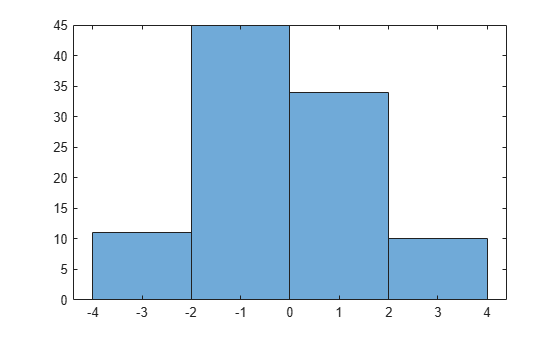# randsrc

Generate random matrix using prescribed alphabet

## Syntax

``out = randsrc``
``out = randsrc(m)``
``out = randsrc(m,n)``
``out = randsrc(m,n,alphabet)``
``out = randsrc(m,n,[alphabet; prob])``
``out = randsrc(m,n,___,seed)``
``out = randsrc(m,n,___,streamhandle)``

## Description

````out = randsrc` generates a random scalar that is either `-1` or `1`, with equal probability.```
````out = randsrc(m)` generates an `m`-by-`m` random bipolar matrix. Each entry independently takes the value `-1` or `1` with equal probability.```
````out = randsrc(m,n)` generates an `m`-by-`n` random bipolar matrix. Each entry independently takes the value `-1` or `1` with equal probability.```

example

````out = randsrc(m,n,alphabet)` generates an `m`-by-`n` matrix, with each entry independently chosen from the entries in the row vector `alphabet`. Each entry in `alphabet` occurs in `out` with equal probability. Duplicate values in `alphabet` are ignored.```
````out = randsrc(m,n,[alphabet; prob])` generates an `m`-by-`n` matrix, with each entry independently chosen from the entries in the row vector `alphabet`. Duplicate values in `alphabet` are ignored. The row vector `prob` lists corresponding probabilities, so that the symbol `alphabet(k)` occurs with probability `prob(k)`, where `k` is any integer between one and the number of columns of `alphabet`. The elements of `prob` must add up to 1.```

example

````out = randsrc(m,n,___,seed)` accepts input combinations from prior syntaxes and a seed value, for initializing the uniform random number generator, `rand`. ```
``` `out = randsrc(m,n,___,streamhandle)` accepts input combinations from prior syntaxes and a random stream handle to generate uniform random noise samples by using `rand`. Providing a random stream handle or using the `reset (RandStream)` function on the default random stream object enables you to generate repeatable noise samples. If you want to generate repeatable noise samples, then either reset the random stream input before calling `randsrc` or use the same seed input. For more information, see `RandStream`.```

## Examples

collapse all

Generate a 10-by-10 matrix from the set of {-3,-1,1,3}.

`out = randsrc(10,10,[-3 -1 1 3])`
```out = 10×10 3 -3 1 1 -1 -1 3 3 -1 -3 3 3 -3 -3 -1 1 -1 -1 3 -3 -3 3 3 -1 3 1 1 3 1 1 3 -1 3 -3 3 -3 1 -3 1 3 1 3 1 -3 -3 -3 3 3 3 3 -3 -3 3 3 -1 -1 3 -1 -1 -3 -1 -1 1 1 -1 3 1 -3 3 1 1 3 -1 -1 1 -1 -3 -1 3 -1 3 3 1 3 1 1 -3 1 -1 -3 3 3 -3 -3 3 -3 -1 -1 1 -1 ```

Plot the histogram. Each of the four possible element values occur with equal probability. Your values might differ.

`histogram(out,[-4 -2 0 2 4])`Generate a matrix in which the likelihood of a -1 or 1 is four times higher than the likelihood of a -3 or 3.

`out = randsrc(10,10,[-3 -1 1 3; 0.1 0.4 0.4 0.1])`
```out = 10×10 -1 -1 -1 -1 1 -1 1 -1 1 3 1 -3 3 3 1 -3 -1 -1 -1 1 -1 -1 -3 -1 -1 3 -1 1 1 -1 1 3 1 -1 1 3 -1 -3 -1 -1 -1 -1 1 -1 -1 -1 -3 -3 1 -1 1 1 1 -1 -3 -1 -1 -1 -1 -1 -1 1 -3 1 -1 -1 3 1 -1 1 1 3 -1 1 -1 3 3 1 1 1 1 -3 -1 1 -1 -1 1 1 1 1 1 -1 1 -1 -1 -1 -3 -1 -3 1 ```

Plot the histogram. Values of -1 and 1 are more likely.

`histogram(out,[-4 -2 0 2 4])`## Input Arguments

collapse all

Size of random bipolar matrix, specified as a scalar. If `n` is specified, then `m` is the row size of the random bipolar matrix.

Data Types: `double`

Column size of random binary matrix, specified as a scalar.

Data Types: `double`

Possible elements of output vector or matrix. If `alphabet` is a row vector, the contents of `alphabet` define which possible elements `randsrc` output. If `alphabet` is a two-row matrix, then the first row is defines the possible elements, and the second row defines the probabilities for each corresponding element in the first row. The elements of the second row must sum to one. If all entries of alphabet are distinct, then the probability distribution is uniform.

Data Types: `double`
Complex Number Support: Yes

Row vector of probabilities that correspond to elements of the corresponding `alphabet` vector.

Data Types: `double`

Seed value for initializing the uniform random number generator, `rand`.

Data Types: `double`

Random stream handle to generate uniform random noise samples by using `rand`. Providing a random stream handle or using the `reset (RandStream)` function on the default random stream object enables you to generate repeatable noise samples. If you want to generate repeatable noise samples, then either reset the random stream input before calling `randsrc` or use the same seed input. For more information, see `RandStream`.

Data Types: `double`

## Output Arguments

collapse all

Random output, returned as a scalar, vector, or matrix. The dimensions of the output are specified by arguments `m` and `n`, otherwise it is a 1-by-1 scalar. The possible elements of the output and their probabilities are specified by `alphabet`, `prob` respectively, otherwise the elements of the output are `-1` and `1`, with equal distribution.

Data Types: `double`

## Version History

Introduced before R2006a